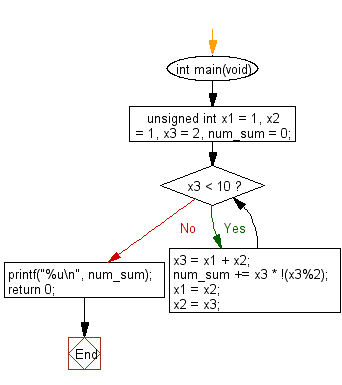﻿ C Program: Sum even-valued in a Fibonacci sequence# C Exercises: Find the sum of the even-valued terms from the terms in the Fibonacci sequence

## C Programming Practice: Exercise-18 with Solution

In mathematics, the Fibonacci numbers, commonly denoted Fn form a sequence, called the Fibonacci sequence, such that each number is the sum of the two preceding ones, starting from 0 and 1. That is, and for n > 1. By starting with 1 and 2, the first 10 terms will be: 1, 2, 3, 5, 8, 13, 21, 34, 55, 89, . . .
Write a C programming to find the sum of the even-valued terms from the terms in the Fibonacci sequence whose values do not exceed one million.

C Code:

``````#include <stdio.h>
int main(void)
{
unsigned int x1 = 1, x2 = 1, x3 = 2, num_sum = 0;
while (x3 < 10) {
x3 = x1 + x2;
num_sum += x3 * !(x3%2);
x1 = x2;
x2 = x3;
}
printf("%u\n", num_sum);
return 0;
}
``````

Sample Output:

```10
```

Flowchart:## C Programming Code Editor:

What is the difficulty level of this exercise?

Test your Programming skills with w3resource's quiz.

﻿

## C Programming: Tips of the Day

What is the difference between printf() and puts() in C?

puts is simpler than printf but be aware that the former automatically appends a newline. If that's not what you want, you can fputs your string to stdout or use printf.

Ref :https://bit.ly/3f2fwcH